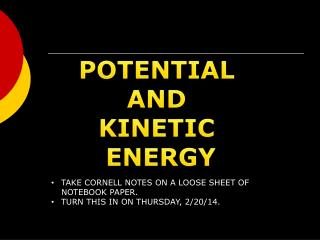DownloadDownload PresentationPOTENTIAL AND KINETIC ENERGY

# POTENTIAL AND KINETIC ENERGY

Download Presentation## POTENTIAL AND KINETIC ENERGY

- - - - - - - - - - - - - - - - - - - - - - - - - - - E N D - - - - - - - - - - - - - - - - - - - - - - - - - - -
##### Presentation Transcript

1. POTENTIAL AND KINETIC ENERGY • TAKE CORNELL NOTES ON A LOOSE SHEET OF NOTEBOOK PAPER. • TURN THIS IN ON THURSDAY, 2/20/14.

2. States of Energy • All forms of energy can be in either of two states: • Potential • Kinetic

3. States of Energy: Kinetic and Potential Energy • Kinetic Energy is energy in motion / action. • Potential Energy is stored energy waiting to be released. Watch this!

4. Kinetic Energy • The energy of motion is called kinetic energy. • The faster an object moves, the morekinetic energy it has. • The greater the mass of a moving object, the more kinetic energy it has. • Kinetic energy depends on both mass and velocity.

5. Potential Energy • Potential Energy is stored energy. • Stored chemically in fuel, the nucleus of atom, and in foods. • Or stored because of the work done on it: • Stretching a rubber band. • Winding a watch. • Pulling back on a bow’s arrow. • Lifting a brick high in the air.

6. Elastic Potential Energy • Energy that is stored due to being stretched or compressed is called elastic potential energy.

7. Gravitational Potential Energy • Potential energy that is dependent on height is called gravitational potential energy.

8. Gravitational Potential Energy • A waterfall, a suspension bridge, and a falling snowflake all have gravitational potential energy.

9. Gravitational Potential Energy • If you stand on a 3-meter diving board, you have 3 times the Gravitational Potential Energy, than you had on a 1-meter diving board.

10. Gravitational Potential Energy • “The bigger they are the harder they fall” is not just a saying. It’s true. Objects with more mass have greater Gravitational Potential Energy. • The formula to find G.P.E. is G.P.E. =Weight X Height.

11. READ ONLY: DO NOT COPY: Kinetic-Potential Energy Conversion Roller coasters work because of the energy that is built into the system. Initially, the cars are pulled mechanically up the tallest hill, giving them a great deal of potential energy. From that highest point, the conversion between potential and kinetic energy powers the cars throughout the entire ride.

12. COPY THIS DIAGRAM AND LABEL MAX KE (kinetic) AND MAX PE (potential) Kinetic vs. Potential Energy At the point of maximum potential energy, the car has minimum kinetic energy.

13. Kinetic-Potential Energy Conversions • As a basketball player throws the ball into the air, various energy conversions take place.

14. COPY THIS DIAGRAM INTO YOUR NOTES: Ball speeds up Ball slows down

15. Questions and Summary • In your questions column, write 3 questions about your notes • In your summary column, write 2-3 sentences explaining what you learned in this PPT.